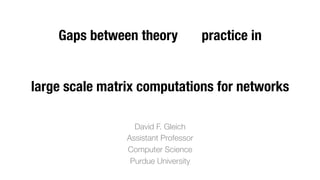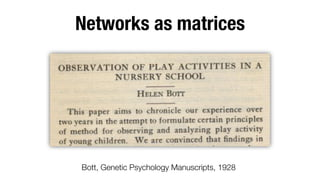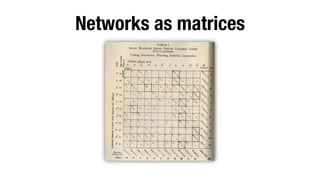Ce diaporama a bien été signalé.
Le téléchargement de votre SlideShare est en cours. ×

# Gaps between the theory and practice of large-scale matrix-based network computations

Publicité
Publicité
Publicité
Publicité
Publicité
Publicité
Publicité
Publicité
Publicité
Publicité
PublicitéChargement dans…3
×

## Consultez-les par la suite

1 sur 28 Publicité

# Gaps between the theory and practice of large-scale matrix-based network computations

I discuss some runtimes for the personalized PageRank vector and how it relates to open questions in how we should tackle these network based measures via matrix computations.

I discuss some runtimes for the personalized PageRank vector and how it relates to open questions in how we should tackle these network based measures via matrix computations.

Publicité
Publicité

### Gaps between the theory and practice of large-scale matrix-based network computations

1. 1. Gaps between theory practice in ! ! ! large scale matrix computations for networks David F. Gleich Assistant Professor Computer Science Purdue University
2. 2. Networks as matrices Bott, Genetic Psychology Manuscripts, 1928
3. 3. Networks as matrices
4. 4. Networks as matrices
5. 5. Networks as matrices A =
6. 6. Everything in the world can be explained by a matrix, and we see how deep the rabbit hole goes The talk ends, you believe -- whatever you want to. Image from rockysprings, deviantart, CC share-alike 6
7. 7. Matrix computations in a red-pill Solve a problem better by exploiting its structure!
8. 8. My research! Models and algorithms for high performance ! A L B Tensormatrix and network computations on data eigenvalues" This proposal is for matchand a power method ing triangles using Network alignment tensor Massive matrix " CH, Y. HOU, AND J. TEMPLETON P methods: maximize Tijk xi xj xk - j0 Triangle j i k k 0 i0 std 2 A L ijk computations subject to kxk2 = 1 AxX b = [x(next) ]i = ⇢ · ( j xk min kAx Tijk xbk+ jk where ! ensures the 2-norm Ax = x SSHOPM method due to " B Fast & Scalable" Network analysis xi ) Kolda and Mayo on multi-threaded IfHuman,proteinsinteraction networks 48,228 and xi (b) Std,j ,= 0.39 cm xk are x and - indicatorsinteraction networks with triangles distributed Yeast protein 257,978 Big tensor T has associated nonzeros triangles data methods too The ~100,000,000,000 architectures 0 0 We work with it implicitly 1 the edges (i, i ), (j, j ), and 8 0
9. 9. One canonical problem PageRank Personalized PageRank Semi-supervised" learning on graphs ( A D ↵ f T ↵A D 1 )x = f adjacency matrix degree matrix regularization “prior” or “givens” Protein function prediction Gene-experiment association Network alignment Food webs
10. 10. One canonical problem ( T ↵A D 1 )x = f Vahab - clustering Karl - clustering Art – prediction Jen - prediction Sebastiano – ranking/centrality
11. 11. An example on a graph PageRank by Google ( ↵A D T 3 5 2 4 1 6 0 B B B B B B @ 1 )x = f 2 31 2 3 2 3 1/6 1/2 0 0 0 0 x1 0 The Model 61/6 0 1/3 0 07C 6x2 7 607 6follow 0 1.61/6 1/2 0 uniformly with 6x 7 607 edges 1/3 0 07C 6 7 6 7 7C 3 6probability , and 7C 6 7 = 6 7 ↵6 0 1/2 0 0 07C 6x4 7 617 61/6 7C 6 7 6 7 4randomly jump 1/3 0 15A 4x5 5 405 2. 1/6 0 1/2 with probability 1/6 , 0 0 1 0 x6 1 we’ll 0 assume everywhere is 0 equally likely non-singular linear system ( < 1), non-negative inverse, works with weights, directed & undirected graphs, weights that don’t sum to less than 1 in each column, …
12. 12. An example on a bigger graph f has a single " one here Newman’s netscience graph 379 vertices 1828 non-zeros “zero” on most of the nodes
13. 13. A special case “one column” or “one node” ( T ↵A D x = column i of ( 1 )x = ei T ↵A D localized solutions 1 ) 1
14. 14. An example on a bigger graph Crawl of ﬂickr from 2006 ~800k nodes, 6M edges, alpha=1/2 0 1.5 error ||xtrue – xnnz||1 10 1 0.5 0 −5 10 −10 10 −15 0 2 4 plot(x) 6 8 10 5 x 10 10 0 10 2 10 4 10 nonzeros 6 10
15. 15. Complexity is complex •  Linear system – O(n3) •  Sparse linear sys. (undir.) – O(m log(m) ) where is a function of latest result on solving SDD systems on graphs •  Neumann series – O(m log( )/log(tol))
16. 16. Forsythe and Liebler, 1950
17. 17. Monte Carlo methods for PageRank K. Avrachenkov et al. 2005. Monte Carlo methods in PageRank Fogaras et al. 2005. Fully scaling personalized PageRank. Das Sarma et al. 2008. Estimating PageRank on graph streams. Bahmani et al. 2010. Fast and Incremental Personalized PageRank Bahmani et al. 2011. PageRank & MapReduce Borgs et al. 2012. Sublinear PageRank Complexity – “O(log |V|)”
18. 18. Gauss-Seidel and Gauss-Southwell Methods to solve A x = b Update x(k+1) = x(k) + ⇢j ej such that [Ax(k+1) ]j = [b]j In words “Relax” or “free” the jth coordinate of your solution vector in order to satisfy the jth equation of your linear system. Gauss-Seidel repeatedly cycle through j = 1 to n Gauss-Southwell use the value of j that has the highest magnitude residual r(k) = b Ax(k)
19. 19. Matrix computations in a red-pill Solve a problem better by exploiting its structure!
20. 20. Gauss-Seidel/Southwell for PageRank w/ access to in-links & degs. PageRankPull (k +1) j = blue node Solve for xj xj(k+1) (k) ↵xa /6 (k) ↵xb /2 (k) ↵xc /3 = fj xj(k+1) w/ access to out-links PageRankPush ↵ X i!j xi(k ) /degi = fj Let b a c j = blue node r(k) = f + ↵AT D 1 (k ) x (k+1) = xj(k) + rj (k +1) =0 then xj Update r(k +1) rj (k (k) ra +1) = ra + ↵rj(k ) /3 (k (k) rb +1) = rb + ↵rj(k ) /3 r (k +1) = r (k) + ↵r (k ) /3 x(k)
21. 21. Python code for PPR Push # main loop! while sumr > eps/(1-alpha):! j = max(r.iteritems, ! key=(lambda x: r[x])! rj = r[j]! x[j] += rj! r[j] = 0! sumr -= rj! deg = len(graph[j])! for i in graph[j]:! if i not in r: r[i] = 0.! r[j] += alpha/deg*rj! sumr += alpha/deg*rj! # initialization ! # graph is a set of sets! # eps is stopping tol! # 0 < alpha < 1! x = dict()! r = dict()! sumr = 0.! for (node,fi) in f.items():! r[node] = fi! sumr += fi! ! If f ≥ 0, this terminates when ||xtrue – xalg||1 <
22. 22. Relaxation methods for PageRank Arasu et al. 2002, PageRank computation and the structure of the web Jeh and Widom 2003, Scaling personalized PageRank McSherry 2005, A uniﬁed framework for PageRank acceleration Andersen et al. 2006, Local PageRank Berkhin 2007, Bookmark coloring algorithm for PageRank Complexity – “O( |E| )”
23. 23. 5 10 Monte Carlo Relaxation 0 10 Sublinear" “in theory” ||xtrue – xalg||1 gap −5 10 Node degree=155 22k node, 2M edge 10−10 Facebook graph gap nnz(A) 10" nnz(A) Number of edges the algorithm touches 4 10 How I’d solve it 6 10 8 10
24. 24. Matrix computations in a red-pill Solve a problem better by exploiting its structure!
25. 25. Some unity? Theorem (Gleich and Kloster, 2013 arXiv:1310.3423)" Consider solving personalized PageRank using the GaussSouthwell relaxation method in a graph with a Zipf-law in the degrees with exponent p=1 and max-degree d, then the work involved in getting a solution with 1-norm error is ⇤ ⇣ work = O (1/") 1 1 ↵ d(log d)2 ⌘ Improve this? * (the paper currently bounds exp(A D-1) ei but analysis yields this bound for PageRank) ** (this bound is not very useful, but it justiﬁes that this method isn’t horrible in theory)
26. 26. There is more structure The one ring. G1 G2 G3 (See C. Seshadhri’s talk for the reference) G4
27. 27. Further open directions Nice to solve Unifying convergence results for Monte Carlo and relaxation on large networks to have provably efﬁcient, practical algs. –  Use triangles? Use preconditioning? A curiosity Is there any reason to use a Krylov method? –  Staple of matrix computations, A ! AVk+1 = Vk Hk with Hk small BIG gap Can we get algorithms with “top k” or “ordering” convergence? –  See Bahmani et al. 2010; Sarkar et al. 2008 (Proximity Search) Important? Are the useful, tractable multi-linear problems on a network? –  e.g. triangles for network alignment; e.g. Kannan’s planted clique problem. Supported by NSF CAREER 1149756-CCF www.cs.purdue.edu/homes/dgleich